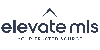Refinement

### 500 Results Found

Maximum number of listings showing. Try narrowing your search results.

#### Search Results

• Status Active

#### 2208 Essex Lane, Colorado Springs, Colorado CO 80909

Bedrooms

Total Baths

2,090 SqFt
0.173 Acresr46108956
38.86504,-104.762672Active
• Status Active

#### 6321 Kildare Drive, Colorado Springs, Colorado CO 80927-9677

Bedrooms

Total Baths

2,197 SqFt
0.103 Acresr46108956
38.922822,-104.655234Active
• Status Active

#### 3889 Mariposa Street, Colorado Springs, Colorado CO 80907-4603

Bedrooms

Total Baths

2,054 SqFt
0.184 Acresr46108956
38.889388,-104.809174Active
• Status Active

#### 9246 Rockingham Drive, Peyton, Colorado CO 80831

Bedrooms

Total Baths

3,883 SqFt
0.550 Acresr46108956
38.973058,-104.627943Active
• Status Active

#### 7652 Straight Shooter View, Colorado Springs, Colorado CO 80923-7579

Bedrooms

Total Baths

2,734 SqFt
0.073 Acresr46108956
38.922792,-104.683796Active
• Status Active

#### 2625 Villa Loma Drive, Colorado Springs, Colorado CO 80917-4038

Bedrooms

Total Baths

2,002 SqFt
0.241 Acresr46108956
38.870156,-104.730907Active
• Status Active

#### 6079 Bennach Grove, Colorado Springs, Colorado CO 80927-4212

Bedrooms

Total Baths

2,542 SqFt
0.124 Acresr46108956
38.921601,-104.661855Active
• Status Active

#### 9720 Avenida Alegre Point, Fountain, Colorado CO 80817-7046

Bedrooms

Total Baths

1,596 SqFt
9 Acresr46108956
38.523321,-104.651267Active
• Status Active

#### 732 E Chestnut Street, Elizabeth, Colorado CO 80107

Bedrooms

Total Baths

1,709 SqFt
0.207 Acresr46108956
39.356502,-104.599598Active
• Status Active

#### Listing Price \$440,000

Bedrooms

Total Baths

1,812 SqFt
0.140 Acresr46108956
38.846928,-104.771399Active
{"action":"https:\/\/search.coloradonewhomespecialists.com\/idx\/results\/listings","hiddenInputs":"<input name=\"start\" type=\"hidden\" value=\"1\" \/>\n<input name=\"hp\" type=\"hidden\" value=\"600000\" \/>\n<input name=\"lp\" type=\"hidden\" value=\"400000\" \/>\n<input name=\"pt\" type=\"hidden\" value=\"1\" \/>\n<input name=\"idxID\" type=\"hidden\" value=\"b035\" \/>\n<input name=\"city[]\" type=\"hidden\" value=\"2079\" \/>\n<input name=\"city[]\" type=\"hidden\" value=\"2305\" \/>\n<input name=\"city[]\" type=\"hidden\" value=\"4254\" \/>\n<input name=\"city[]\" type=\"hidden\" value=\"6758\" \/>\n<input name=\"city[]\" type=\"hidden\" value=\"7542\" \/>\n<input name=\"city[]\" type=\"hidden\" value=\"60485\" \/>\n<input name=\"city[]\" type=\"hidden\" value=\"7632\" \/>\n<input name=\"city[]\" type=\"hidden\" value=\"8660\" \/>\n<input name=\"city[]\" type=\"hidden\" value=\"9780\" \/>\n<input name=\"city[]\" type=\"hidden\" value=\"9783\" \/>\n<input name=\"city[]\" type=\"hidden\" value=\"9915\" \/>\n<input name=\"city[]\" type=\"hidden\" value=\"10870\" \/>\n<input name=\"city[]\" type=\"hidden\" value=\"12081\" \/>\n<input name=\"city[]\" type=\"hidden\" value=\"12374\" \/>\n<input name=\"city[]\" type=\"hidden\" value=\"14124\" \/>\n<input name=\"city[]\" type=\"hidden\" value=\"14215\" \/>\n<input name=\"city[]\" type=\"hidden\" value=\"14355\" \/>\n<input name=\"city[]\" type=\"hidden\" value=\"59462\" \/>\n<input name=\"city[]\" type=\"hidden\" value=\"16730\" \/>\n<input name=\"city[]\" type=\"hidden\" value=\"16928\" \/>\n<input name=\"city[]\" type=\"hidden\" value=\"19016\" \/>\n<input name=\"city[]\" type=\"hidden\" value=\"19510\" \/>\n<input name=\"city[]\" type=\"hidden\" value=\"21190\" \/>\n<input name=\"city[]\" type=\"hidden\" value=\"25618\" \/>\n<input name=\"city[]\" type=\"hidden\" value=\"28186\" \/>\n<input name=\"city[]\" type=\"hidden\" value=\"31038\" \/>\n<input name=\"city[]\" type=\"hidden\" value=\"7\" \/>\n<input name=\"city[]\" type=\"hidden\" value=\"35244\" \/>\n<input name=\"city[]\" type=\"hidden\" value=\"35525\" \/>\n<input name=\"city[]\" type=\"hidden\" value=\"36117\" \/>\n<input name=\"city[]\" type=\"hidden\" value=\"36348\" \/>\n<input name=\"city[]\" type=\"hidden\" value=\"42074\" \/>\n<input name=\"city[]\" type=\"hidden\" value=\"42076\" \/>\n<input name=\"city[]\" type=\"hidden\" value=\"46280\" \/>\n<input name=\"city[]\" type=\"hidden\" value=\"49035\" \/>\n<input name=\"city[]\" type=\"hidden\" value=\"52548\" \/>\n<input name=\"city[]\" type=\"hidden\" value=\"52928\" \/>\n<input name=\"srt\" type=\"hidden\" value=\"newest\" \/>\n","maxPerPage":"0","per":"10","currentPage":1,"lastPage":50,"path":"https:\/\/search.coloradonewhomespecialists.com\/idx\/results\/listings?start=1&hp=600000&lp=400000&per=10&pt=1&idxID=b035&city%5B%5D=2079&city%5B%5D=2305&city%5B%5D=4254&city%5B%5D=6758&city%5B%5D=7542&city%5B%5D=60485&city%5B%5D=7632&city%5B%5D=8660&city%5B%5D=9780&city%5B%5D=9783&city%5B%5D=9915&city%5B%5D=10870&city%5B%5D=12081&city%5B%5D=12374&city%5B%5D=14124&city%5B%5D=14215&city%5B%5D=14355&city%5B%5D=59462&city%5B%5D=16730&city%5B%5D=16928&city%5B%5D=19016&city%5B%5D=19510&city%5B%5D=21190&city%5B%5D=25618&city%5B%5D=28186&city%5B%5D=31038&city%5B%5D=7&city%5B%5D=35244&city%5B%5D=35525&city%5B%5D=36117&city%5B%5D=36348&city%5B%5D=42074&city%5B%5D=42076&city%5B%5D=46280&city%5B%5D=49035&city%5B%5D=52548&city%5B%5D=52928&srt=newest"}The real estate listing information and related content displayed on this site is provided exclusively for consumers' personal, non-commercial use and may not be used for any purpose other than to identify prospective properties consumers may be interested in purchasing. Any offer of compensation is made only to Participants of the PPMLS. This information and related content is deemed reliable but is not guaranteed accurate by the Pikes Peak REALTOR® Services Corp.

Information Last Updated: Monday, October 3rd, 2022
Data services provided by IDX Broker